# The Bigyrate Diminished Rhombicosidodecahedron

The bigyrate diminished rhombicosidodecahedron is the 79th Johnson solid, or J79 for short. It has 52 faces (1 decagon, 11 pentagons, 25 squares, and 15 triangles), 105 edges, and 55 vertices. It is obtained by cutting off a pentagonal cupola segment from the rhombicosidodecahedron and gyrating two oblique pentagonal cupola segments.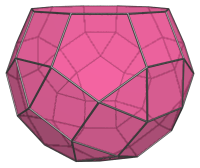The bigyrate diminished rhombicosidodecahedron closely resembles the diminished rhombicosidodecahedron (J76), but can be distinguished from the latter by telltale pairs of adjacent squares, which are not present in J76: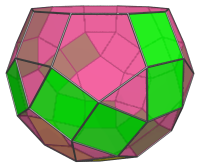It can also be distinguished from also very similar J77 and J78 by the strip of 3 adjacent squares unique to the metabigyrates of the rhombicosidodecahedron, highlighted in yellow below: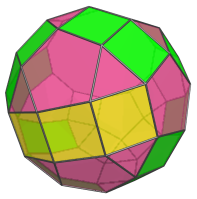These pairs of adjacent squares and strip of 3 adjacent squares occur because of the gyrated orientation of the two pentagonal cupola segments highlighted below: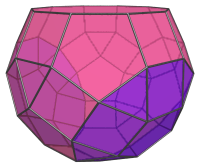## Projections

Here are some views of the bigyrate diminished rhombicosidodecahedron from various angles. For ease of reference, we highlight the strip of 3 squares in yellow.

Projection Description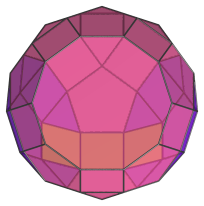Top view.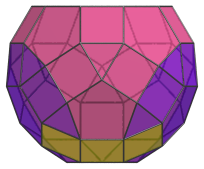Front view.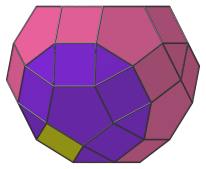Side view.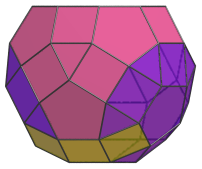Oblique side view.

## Coordinates

The Cartesian coordinates of the bigyrate diminished rhombicosidodecahedron with edge length 2 are:

• (±(2+φ), ±(1+φ), 0)
• (±(1+φ), 0, −(2+φ))
• (0, 2+φ, ±(1+φ))
• (0, −(2+φ), 1+φ)
• (±1, ±(1+2φ), ±1)
• (±(1+2φ), ±1, −1)
• (±1, 1, ±(1+2φ))
• (±1, −1, 1+2φ)
• (±(1+φ), ±2φ, ±φ)
• (±2φ, ±φ, −(1+φ))
• (±φ, 1+φ, ±2φ)
• (±φ, −(1+φ), 2φ)
• (−(8φ+1)/5, ±1, (6φ+7)/5)
• (−(8φ+6)/5, ±φ, (7+φ)/5)
• (−(13φ+1)/5, 0, (φ+2)/5)
• (±1, −(6φ+7)/5, −(8φ+1)/5)
• (±φ, −(7+φ)/5, −(8φ+6)/5)
• (0, −(φ+2)/5, −(13φ+1)/5)

where φ=(1+√5)/2 is the Golden Ratio.

Last updated 21 May 2019.